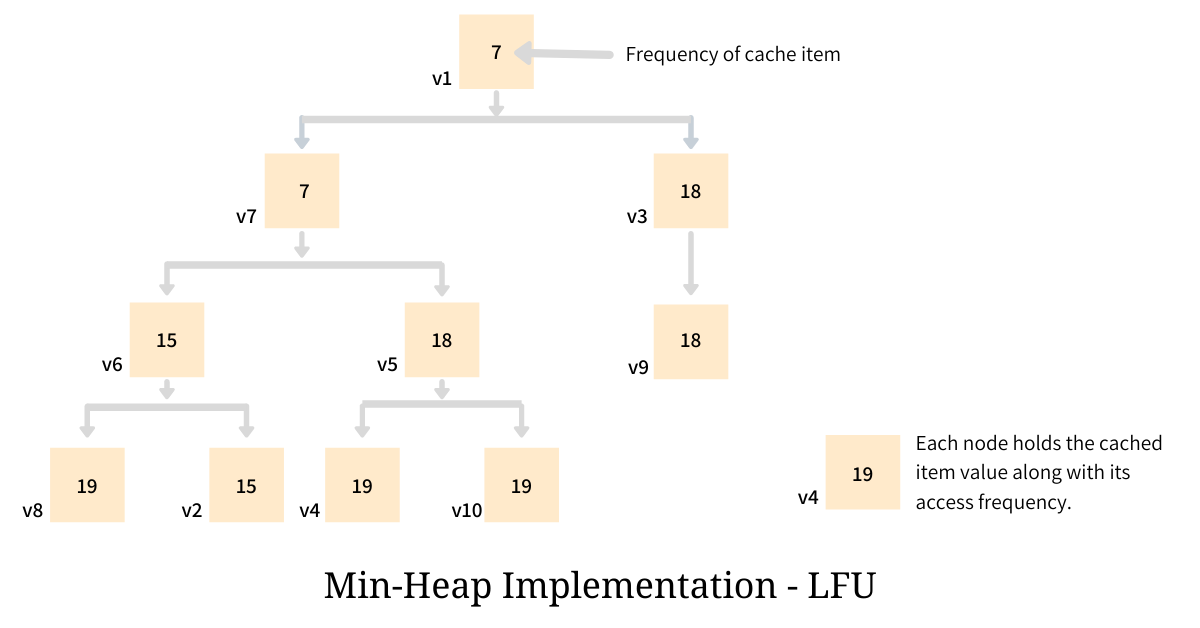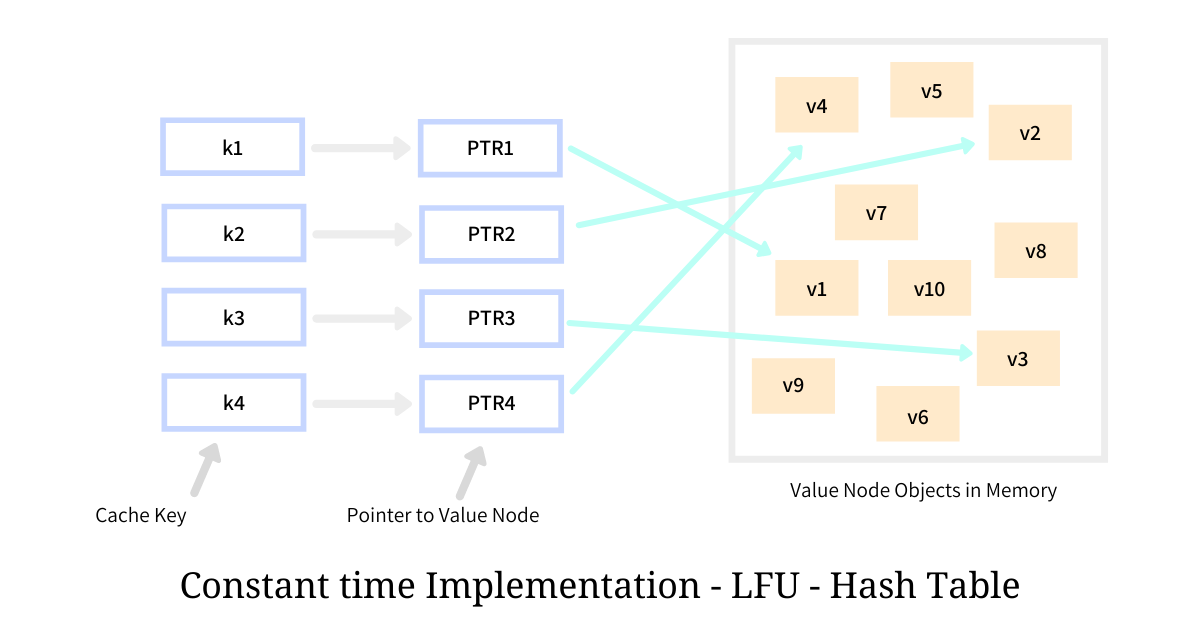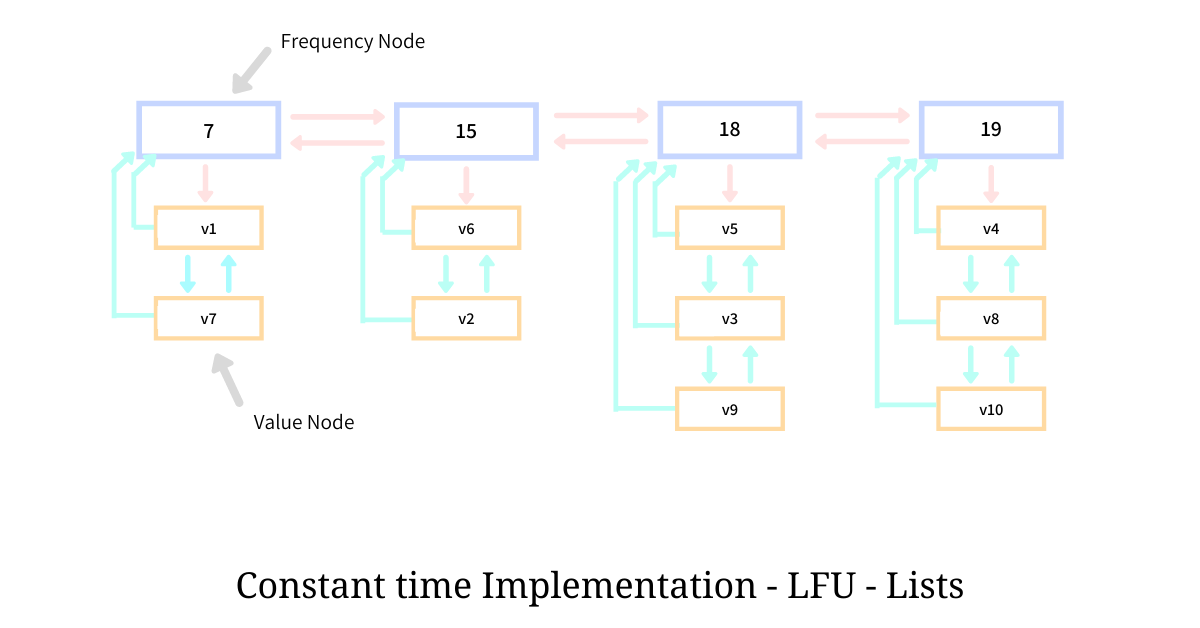Registrations for the November 2021 System Design Cohort are now open# Constant Time LFU

Published on 23rd Aug 2020

A common strategy to make any system super-performant is Caching. Almost all software products, operating at scale, have multiple layers of caches in their architectures. Caching, when done right, does wonder to the response time and is one of the main reasons why products work so well at a massive scale. Cache engines are limited by the amount of memory available and hence once it gets full the engine has to decide which item should be evicted and that is where an eviction algorithm, like LFU and LRU. kicks in.

LRU (Least Recently Used) cache eviction strategy is one of the most popular strategies out there. LFU (Least Frequently Used) strategy fares well in some use cases but a concern with its most popular implementation, where it uses a min-heap, is that it provides a running time complexity of `O(log n)` for all the three operations - insert, update and delete; because of which, more often than not, it is replaced with a sub-optimal yet extremely fast `O(1)` LRU eviction scheme.

In this essay, we take a look at Constant Time LFU implementation based on the paper An O(1) algorithm for implementing the LFU cache eviction scheme by Prof. Ketan Shah, Anirban Mitra and Dhruv Matani, where instead of using a min-heap, it uses a combination of doubly-linked lists and hash table to gain a running time complexity of `O(1)` for all the three core operations.

# The LFU cache eviction strategy

LFU, very commonly, is implemented using a min-heap which is organized as per the frequency of access of each element. Each element of this heap holds a pair - cached value and the access frequency; and is structured in order of this frequency such that the cached value with the minimum access frequency sits at the top, making it quick to identify the element to be evicted.Although the identification of the element to be evicted is quick, but in order for the heap to maintain its property - element with lowest access frequency be at the top - it demands a rebalance, and this rebalancing process has a running complexity of `O(log n)`. To make things worse, rebalancing is required every single time the frequency of an item is changed; which means that in the cache that implements LFU, every time an item is either inserted, accessed or evicted, a rebalance is required - making all the three core operations to have the time complexity of `O(log n)`.

# Constant time implementation

The LFU cache can be implemented with `O(1)` complexity for all the three operations by using one Hash Table and a bunch of Doubly Linked Lists. As stated by the RUM Conjecture, in order to get a constant time reads and updates operations, we have to make a compromise with memory utilization. This is exactly what we observe in this implementation.

## The Hash Table

The Hash Table stores the mapping of the cached key to the Value Node holding the cached value. The value against the key is usually a pointer to the actual Value Node. Given that the lookup complexity of the hash table is `O(1)`, the operation to access the value given the key from this Hash Table could be accomplished in constant time.The illustration above depicts that the Hash Table holding cache keys `k1`, `k2`, etc are mapped to the nodes holding the values `v1` and `v2` through direct pointers. The nodes are allocated on the heap using dynamic allocation, hence are a little disorganized. The Value Node to which the key maps to, not only hold the cached value, but it also holds a few pointers pointing to different entities in the system, enabling constant-time operations.

This implementation of LFU requires us to maintain one Doubly Linked List of frequencies, called `freq_list`, holding one node for each unique frequency spanning all the caches values. This list is kept sorted on the frequency the node represents such that, the node representing the lowest frequency is on the one end while the node representing the highest frequency is at the other.

Every Frequency Node holds the frequency that it represents in the member `freq` and the usual `next` and `prev` pointers pointing to the adjacent Frequency Nodes; it also keeps a `values_ptr` which points to another doubly-linked list holding Value Nodes (referred in the hash table) having the same access frequency `freq`.The overall schematic representation of doubly-linked lists and its arrangement is as shown in the illustration above. The doubly-linked list holding Frequency Nodes is arranged horizontally while the list holding the Value Nodes is arranged vertically, for clearer view and understanding.

Since the cached values `v1` and `v7` both have been accessed `7` times, they both are chained in a doubly-linked list and are hooked with the Frequency Node representing the frequency of `7`. Similarly, the Value Nodes holding values `v5`, `v3`, and `v9` are chained in another doubly-linked list and are hooked with the Frequency Node representing the frequency of `18`.

The Value Node contains the cached value in member `data`, along with the usual `next` and `prev` pointers pointing to the adjacent Value Nodes in the list. It also holds a `freq_pointer` pointing back to the Frequency Node to which the list if hooked at. Having all of these pointers helps us ensure all the three operations happen in constant time.

Now that we have put all the necessary structures in place, we take a look at the 3 core operations along with their pseudo implementation.

## Adding value to the cache

Adding a new value to the cache is a relatively simpler operation that requires a bunch of pointer manipulations and does the job with a constant time running complexity. While inserting the value in the cache, we first check the existence of the key in the table, if the key is already present and we try to put it again the function raises an error. Then we ensure the presence of the Frequency Node representing the frequency of `1`, and in the process, we might also need to create a new frequency node also. Then we wrap the value in a Value Node and adds it to the `values_list` of this Frequency Node; and at last, we make an entry in the table acknowledging the completion of the caching process.

``````def add(key: str, value: object):
# check if the key already present in the table,
# if so then return the error
if key in table:

# create the Value Node out of value
# holding all the necessary pointers
value_node = make_value_node(value)

if first_frequency_node.freq != 1:
# since the first node in the freq_list does not represent
# frequency of 1, we create a new node
first_frequency_node = make_frequency_node(1)

# update the `freq_list` that holds all the Frequency Nodes
# such that the first node represents the frequency 1
# and other list stays as is.

# Value Node points back to the Frequency Node to which it belongs
value_node.freq_pointer = first_frequency_node

# add the Value Node in `first_frequency_node`

# update the entry in the hash table
table[key] = value_node
``````

As seen in the pseudocode above the entire procedure to add a new value in the cache is a bunch of memory allocation along with some pointer manipulations, hence we observe that the running complexity of `O(1)` for this operation.

## Evicting an item from the cache

Eviction, similar to insertion, is a trivial operation where we simply pick the frequency node with lowest access frequency (the first node in the `freq_list`) and remove the first Value Node present in its `values_list`. Since the entire eviction also requires pointer manipulations, it also exhibits a running complexity of `O(1)`.

``````def evict():
second_value_node = first_value_node.next

# make the second element as first

# ensure second node does not have a dangling prev link
second_value_node.prev = None

# delete the first element
delete_value_node(first_value_node)

# if the Frequency Node after eviction does not hold
# any values we get rid of it
``````

## Getting a value from the cache

Accessing an item from the cache has to be the most common operation of any cache. In the LFU scheme, before returning the cached value, the engine also has to update its access frequency. Ensuring the change in access frequency of one cached value does not require some sort of rebalancing or restructuring to maintain the integrity, is what makes this implementation special.

The engine first makes a get call to the Hash Table to check that the key exists in the cache. Before returning the cached value from the retrieved Value Node, the engine performs the following operations - it accesses the Frequency Node and its sibling corresponding to the retrieved Value Node. It ensures that the frequency of the sibling is 1 more than that of the Frequency Node; if not it creates the necessary Frequency Node and place it as the new sibling. The Value Node then changes its affinity to this sibling Frequency Node so that it correctly matches the access frequency. In the end, the back pointer from the Value Node to the new Frequency Node is set and the value is returned.

``````def get(key: str) -> object:
# get the Value Node from the hash table
value_node = table.get(key, None)

# if the value does not exist in the cache then return an error
if not value_node:
raise KeyNotFoundError

# we get the Frequency Node from the Value Node using the
# freq_pointer member.
frequency_node = value_node.freq_pointer

# we also get the next Frequency Node to the current
# Frequency Node so that we could make a call about
# the need to create a new node.
next_frequency_node = frequency_node.next

if next_frequency_node.freq != frequency_node.freq + 1:
# create a new Frequency Node
new_frequency_node = make_frequency_node(frequency_node.freq + 1)

# place the Frequency Node at the correct position in the list
frequency_node.next = new_frequency_node
new_frequency_node.prev = frequency_node

next_frequency_node.prev = new_frequency_node
new_frequency_node.next = frequency_node

# going forward we call the new Frequency Node as next
# because it represents the the next Frequency Node
next_frequency_node = new_frequency_node

# we add the Value Node in the nex

# we change the parent and adjecent nodes to this Value Nodes
value_node.freq_pointer = next_frequency_node
value_node.next = None
value_node.prev = next_frequency_node.values.last

# if the Frequency Node has no elements then deleting the node
# so as to avoid the memory leak
if len(frequency_node.values) == 0:
delete_frequency_node(frequency_node)

# returning the value
return value_node.value
``````

Again, since this operation also only deals with pointer manipulations through direct pointers, the running time complexity of this operation is also constant time. Thus we see the Constant Time LFU implementation where the necessary time complexity is achieved by using Hash Tables and Doubly-Linked Lists.

# References

If my work adds value, consider supporting me## 2000+ Subscribers

If you like what you read subscribe you can always subscribe to my newsletter and get the post delivered straight to your inbox. I write essays on various engineering topics and share it through my weekly newsletter 👇

# Other articles that you might likeFully Persistent Arrays

Persistent Data Structures allow us to hold multiple versions of a data structure at any given insta...

14th FebApproximate Count-Distinct using Flajolet Martin Algorithm

Measuring distinct elements from a stream of values is one of the most common utilities that finds i...

6th DecConsistent Hashing

Consistent Hashing is one of the most sought after techniques when it comes to designing highly scal...

24th MayIsolation Forest Algorithm for Anomaly Detection

Anomaly detection is an age-old problem and in this article, we dive deep into an unsupervised algor...

28th Jan